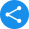# RD Sharma Solutions for Class 6 to 12 Mathematics

## RD Sharma Class 6 to 12 Maths Solutions - Free PDF Download

RD Sharma Solutions will put to rest all your worries and help a student excel in Mathematics. If it is a subject that students are struggling with, these solutions of RD Sharma will guide them step-by-step through the various chapters prescribed in CBSE textbooks. Catered to Class 6 to 12 students, RD Sharma Maths Solutions is categorized according to standards 6 to 12. The Class 6 to 12 RD Sharma Math Solution PDF provides step-by-step solutions to unsolved problems for Class 9 Math textbooks and includes chapters like the Number System, Rationalization, Algebraic Identities, Heron's Formula, and others, while the Class 10 RD Sharma Math Solution PDF provides step-by-step solutions to unsolved problems for Class 10 Math textbooks with chapters related to the Real Numbers, Polynomials, Triangles, Statistics, Quadratic Equations, Circles, Constructions, and others. Chapters on coordinate geometry and congruent triangles are included for both the Class 9 and Class 10 PDFs and if one is confused about the difference between construction and coordinates, you can rest assured that they'll be taken on an extensive and interactive trip through the simplified concepts in these PDFs. The RD Sharma Book Solutions PDF is available for all students across the nation as a free download which can be obtained by visiting Vedantu.com. All you need is basic internet access.

### RD Sharma Solutions for Class 6 to 12 Mathematics

1. Who is RD Sharma?

A. Dr Ravi Dutt Sharma, renowned as RD Sharma, was a Mathematics teacher and author of 25 reference books. His name evokes much awe for all the subjects he taught. He had written several Mathematics books for Elementary, High schools, and Graduate level students. These books became quite popular among the ICSE and CBSE students for its comprehensive material. Without his books, the exam preparation will remain incomplete.

2. Why is RD Sharma renowned for?

A. RD Sharma Solutions are considered as the best study materials. Students who fear Maths should definitely opt for RD Sharma solutions as using these will put to rest all of their worries and help them excel in Mathematics. These RD Sharma solutions will guide them step-by-step through the various chapters prescribed in CBSE/ NCERT textbooks. These Maths books and the solutions are catered to all Class 6 to 12 students. The Class 6 to 12 RD Sharma Maths Solutions PDFs offered by Vedantu provide step-wise solutions to the problems asked in the exercises of the textbooks’ chapters. Class 9 RD Sharma solutions include chapters such as the Number System, Rationalization, Algebraic Identities, Heron's Formula etc while the Class 10 RD Sharma Math Solution PDF provides step-by-step solutions to the problems for the chapters related to the Real Numbers, Polynomials, Triangles, Statistics, Quadratic Equations, Circles, Constructions, and others. Chapters on coordinate geometry and congruent triangles are also being included for both the Class 9 and Class 10 PDFs. If you have doubts, you should always opt for RD Sharma books and solutions so that you can rest assured that all your doubts are taken care of in a simplified and concise manner.

3. Does Vedantu provide solutions for RD Sharma Maths textbook?

A. Vedantu provides solutions for RD Sharma Maths textbooks for Class 6 to Class 12 students. These solutions are available in downloadable PDF format on the website and the mobile app as well. From finding applications in daily life to advanced principles instilled in university projects, studying the RD Sharma free PDF solutions will allow students to develop a strong foundation in Maths. Maths, which sounds fearful and challenging to many, will no longer be a daunting subject when a student refers to an RD Sharma Exercise and the solutions to it. Guided with step-by-step solutions along with diagrams, every concept is explained in the easy-to-understand format and this helps students a great deal in preparing for their Class 9 and Class 10 board exams. The Class 10 RD Sharma solutions PDF file includes 16 chapters from prescribed Class 10 Math books while the free Class 9 PDF consists of 25 chapters in total which cover the entire Class 9 and Class 10 CBSE Mathematics curriculum.

4. What are the specialities of the RD Sharma Maths textbooks?

A. The more you solve and practice different types of Maths problems, the faster you can solve more difficult problems with more accuracy that are asked on the board and other competitive exams. Besides the traditional ways of solving problems, RD Sharma Solutions also aimed on learning various Mathematics tricks and shortcuts for quick and easy calculations. These tricks and shortcuts will definitely change your approach towards modern Mathematics and help you to grow your interest in this subject.

The students can not score well in Mathematics by just reading and memorizing the concepts and formulas. The one and the only way to score high is just practice and more practice. Hence, RD Sharma books contain ample amounts of questions along with their solutions, shortcut techniques, and in-depth explanations. The students are required to go through and solve the problems given in the RD Sharma Solutions thoroughly before the final exams to score well and enhance their problem-solving abilities. These solutions are primarily designed for CBSE students and are based on the latest CBSE curriculum as per the specified guidelines.

Share this with your friendsShare